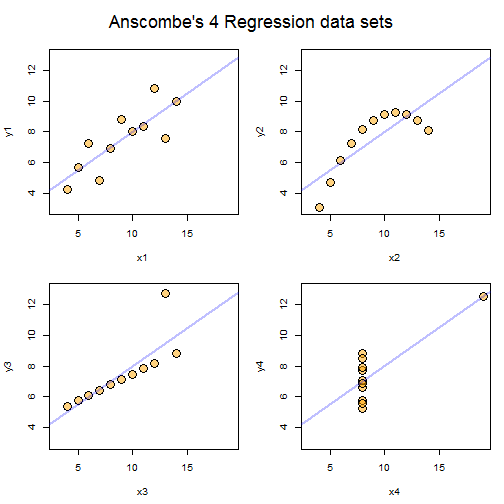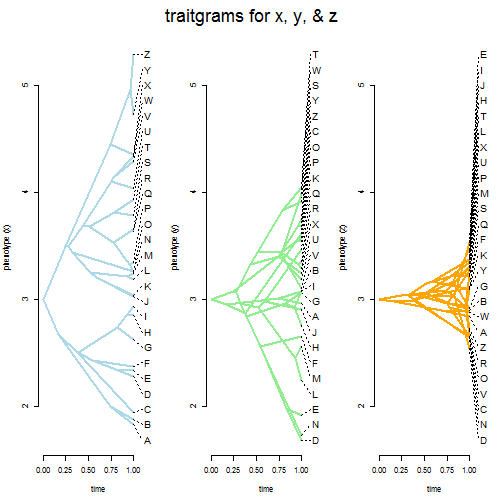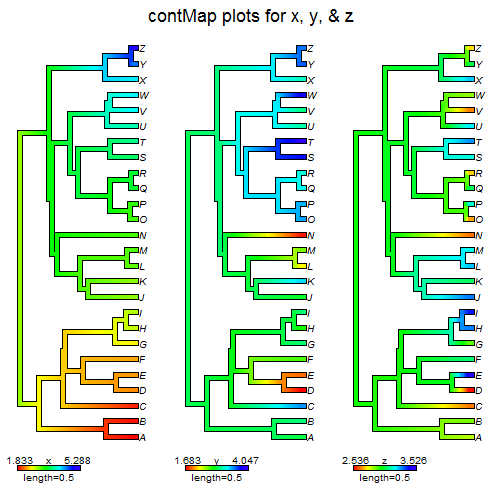## Tuesday, October 17, 2017

### Phylogenetic 'Anscombe' datasets

Since phytools contains a range of different methods for visualizing trait evolution on trees, I have been thinking a bit about the value of plotting our data, rather than just fitting sophisticated models & reporting the results (as is quite common).

The importance of doing this kind of graphing in statistics is now fairly well appreciated; however, this has not always been the case. For instance, in 1973 Francis Anscombe illustrated the importance of graphing with his famous 'quartet,' published in a paper designed to counter the impression among statisticians that “numerical calculations are exact, but graphs are rough” (source).

The Anscombe quartet is a set of four bivariate datasets that are virtually identical in any descriptive statistic (means, regression coefficients, F-values, r2, P-values, and so on), but that when graphed reveal clearly different patterns of covariation between the x & y.

This is what I mean. [Note that all of the following is modified, substantially - but not in substance, from the documentation of the datasets package.]

``````library(datasets)
attach(anscombe)
anscombe
``````
``````##    x1 x2 x3 x4    y1   y2    y3    y4
## 1  10 10 10  8  8.04 9.14  7.46  6.58
## 2   8  8  8  8  6.95 8.14  6.77  5.76
## 3  13 13 13  8  7.58 8.74 12.74  7.71
## 4   9  9  9  8  8.81 8.77  7.11  8.84
## 5  11 11 11  8  8.33 9.26  7.81  8.47
## 6  14 14 14  8  9.96 8.10  8.84  7.04
## 7   6  6  6  8  7.24 6.13  6.08  5.25
## 8   4  4  4 19  4.26 3.10  5.39 12.50
## 9  12 12 12  8 10.84 9.13  8.15  5.56
## 10  7  7  7  8  4.82 7.26  6.42  7.91
## 11  5  5  5  8  5.68 4.74  5.73  6.89
``````
``````fits<-list()
for(i in 1:4){
model<-paste("y",i,"~x",i,sep="")
cat(paste("Model",i,"\n--------",model,"--------\n"))
fits[[i]]<-lm(model)
cat("\n")
print(coef(fits[[i]]))
print(anova(fits[[i]]))
cat("\n-----------------------\n\n")
}
``````
``````## Model 1
## -------- y1~x1 --------
##
## (Intercept)          x1
##   3.0000909   0.5000909
## Analysis of Variance Table
##
## Response: y1
##           Df Sum Sq Mean Sq F value  Pr(>F)
## x1         1 27.510 27.5100   17.99 0.00217 **
## Residuals  9 13.763  1.5292
## ---
## Signif. codes:  0 '***' 0.001 '**' 0.01 '*' 0.05 '.' 0.1 ' ' 1
##
## -----------------------
##
## Model 2
## -------- y2~x2 --------
##
## (Intercept)          x2
##    3.000909    0.500000
## Analysis of Variance Table
##
## Response: y2
##           Df Sum Sq Mean Sq F value   Pr(>F)
## x2         1 27.500 27.5000  17.966 0.002179 **
## Residuals  9 13.776  1.5307
## ---
## Signif. codes:  0 '***' 0.001 '**' 0.01 '*' 0.05 '.' 0.1 ' ' 1
##
## -----------------------
##
## Model 3
## -------- y3~x3 --------
##
## (Intercept)          x3
##   3.0024545   0.4997273
## Analysis of Variance Table
##
## Response: y3
##           Df Sum Sq Mean Sq F value   Pr(>F)
## x3         1 27.470 27.4700  17.972 0.002176 **
## Residuals  9 13.756  1.5285
## ---
## Signif. codes:  0 '***' 0.001 '**' 0.01 '*' 0.05 '.' 0.1 ' ' 1
##
## -----------------------
##
## Model 4
## -------- y4~x4 --------
##
## (Intercept)          x4
##   3.0017273   0.4999091
## Analysis of Variance Table
##
## Response: y4
##           Df Sum Sq Mean Sq F value   Pr(>F)
## x4         1 27.490 27.4900  18.003 0.002165 **
## Residuals  9 13.742  1.5269
## ---
## Signif. codes:  0 '***' 0.001 '**' 0.01 '*' 0.05 '.' 0.1 ' ' 1
##
## -----------------------
``````

Now let's plot them:

``````par(mfrow=c(2,2),mar=c(4.6,4.1,1.1,1.1),oma=c(0,0,3,0))
for(i in 1:4){
model<-paste("y",i,"~x",i,sep="")
plot(as.formula(model),pch=21,
bg=phytools::make.transparent("orange",0.5),cex=2,xlim=c(3,19),
ylim=c(3,13))
abline(fits[[i]],lwd=2,col=phytools::make.transparent("blue",0.25))
}
mtext("Anscombe's 4 Regression data sets",outer=TRUE,cex=1.5,line=0.5)
``````So what of the relevance to phylogenetic data? Well, we could easily imagine a scenario in which very different character patterns could result in the same fitted evolutionary model. For instance, take the following tree & three continuous characters, x, y, & z:

``````library(phytools)
library(geiger)
tree
``````
``````##
## Phylogenetic tree with 26 tips and 25 internal nodes.
##
## Tip labels:
##  A, B, C, D, E, F, ...
##
## Rooted; includes branch lengths.
``````
``````data.frame(x,y,z)
``````
``````##          x        y        z
## A 1.833108 2.962696 2.835180
## B 1.872561 3.073251 2.898368
## C 1.936456 3.614328 2.647927
## D 2.279403 1.682714 2.535930
## E 2.333815 1.917306 3.525612
## F 2.368385 2.652735 3.112808
## G 2.624223 3.059308 2.917372
## H 2.851292 2.755371 3.364408
## I 2.931033 3.072902 3.464446
## J 3.027722 2.899132 3.366387
## K 3.035856 3.463041 3.092368
## L 3.180211 2.238550 3.301345
## M 3.238953 2.558549 3.225307
## N 3.259976 1.723714 2.641809
## O 3.262605 3.580004 2.657079
## P 3.339634 3.540232 3.227204
## Q 3.632080 3.349932 3.112958
## R 3.653154 3.283649 2.703744
## S 3.783546 3.916703 3.213564
## T 3.935576 4.046783 3.341507
## U 4.037169 3.180505 3.264513
## V 4.291665 3.100641 2.648084
## W 4.333509 3.990103 2.874010
## X 4.350045 3.241618 3.293307
## Y 4.724357 3.747172 3.090894
## Z 5.288439 3.679698 2.722883
``````

If we fit a Brownian model using geiger's `fitContinuous` function we will find that all three have identical Brownian rates, ancestral state values, and even likelihoods!

``````fitContinuous(tree,x)
``````
``````## GEIGER-fitted comparative model of continuous data
##  fitted 'BM' model parameters:
##  sigsq = 0.499997
##  z0 = 3.000000
##
##  model summary:
##  log-likelihood = -16.681907
##  AIC = 37.363814
##  AICc = 37.885553
##  free parameters = 2
##
## Convergence diagnostics:
##  optimization iterations = 100
##  failed iterations = 0
##  frequency of best fit = 1.00
##
##  object summary:
##  'lik' -- likelihood function
##  'bnd' -- bounds for likelihood search
##  'res' -- optimization iteration summary
##  'opt' -- maximum likelihood parameter estimates
``````
``````fitContinuous(tree,y)
``````
``````## GEIGER-fitted comparative model of continuous data
##  fitted 'BM' model parameters:
##  sigsq = 0.499988
##  z0 = 3.000002
##
##  model summary:
##  log-likelihood = -16.681672
##  AIC = 37.363344
##  AICc = 37.885083
##  free parameters = 2
##
## Convergence diagnostics:
##  optimization iterations = 100
##  failed iterations = 0
##  frequency of best fit = 1.00
##
##  object summary:
##  'lik' -- likelihood function
##  'bnd' -- bounds for likelihood search
##  'res' -- optimization iteration summary
##  'opt' -- maximum likelihood parameter estimates
``````
``````fitContinuous(tree,z)
``````
``````## GEIGER-fitted comparative model of continuous data
##  fitted 'BM' model parameters:
##  sigsq = 0.500000
##  z0 = 3.000000
##
##  model summary:
##  log-likelihood = -16.681969
##  AIC = 37.363938
##  AICc = 37.885677
##  free parameters = 2
##
## Convergence diagnostics:
##  optimization iterations = 100
##  failed iterations = 0
##  frequency of best fit = 1.00
##
##  object summary:
##  'lik' -- likelihood function
##  'bnd' -- bounds for likelihood search
##  'res' -- optimization iteration summary
##  'opt' -- maximum likelihood parameter estimates
``````

However, if we visualize our data we shall see that they show strikingly different patterns. To do this I'll use the function `phenogram` from the phytools package:

``````par(mfrow=c(1,3),mar=c(5.1,4.1,1.1,1.1),oma=c(0,0,3,0))
labels<-setNames(seq(min(c(x,y,z)),max(c(x,y,z)),
by=diff(range(c(x,y,z)))/(Ntip(tree)-1)),
names(sort(x)))
label.pos=labels,colors="lightblue",fsize=1.2,
ylab="phenotype (x)")
labels<-setNames(seq(min(c(x,y,z)),max(c(x,y,z)),
by=diff(range(c(x,y,z)))/(Ntip(tree)-1)),
names(sort(y)))
label.pos=labels,colors="lightgreen",fsize=1.2,
ylab="phenotype (y)")
labels<-setNames(seq(min(c(x,y,z)),max(c(x,y,z)),
by=diff(range(c(x,y,z)))/(Ntip(tree)-1)),
names(sort(z)))
label.pos=labels,colors="orange",fsize=1.2,
ylab="phenotype (z)")
mtext("traitgrams for x, y, & z",outer=TRUE,cex=1.5,line=0.5)
``````In one case (x), we have data that are highly phylogenetically structured, at the other extreme (z), our data seem to have very little phylogenetic structure at all, and in the middle (y) we see an intermediate degree of phylogenetic patterning.

We'll get the same impression if we use a `contMap` style plot:

``````par(mfrow=c(1,3),oma=c(0,0,3,0))
obj<-contMap(tree,x,plot=FALSE)
plot(obj,xlim=c(-0.1,1.2),leg.txt="x",fsize=1.2)
obj<-contMap(tree,y,plot=FALSE)
plot(obj,xlim=c(-0.1,1.2),leg.txt="y",fsize=1.2)
obj<-contMap(tree,z,plot=FALSE)
plot(obj,xlim=c(-0.1,1.2),leg.txt="z",fsize=1.2)
mtext("contMap plots for x, y, & z",outer=TRUE,cex=1.5,line=0.5)
``````Unlike the previous plot, here I have not used a consistent trait scale to make the lack of phylogenetic structure in z more apparent.

Of course, if we measured even a relatively simple diagnostic such as phylogenetic signal for the two datasets we would find that they are not at all alike!

``````phylosig(tree,x)
``````
``````##  2.077251
``````
``````phylosig(tree,y)
``````
``````##  1.013159
``````
``````phylosig(tree,z)
``````
``````##  0.207328
``````

You too can generate your own phylogenetic Anscombe datasets. I made mine as follows:

``````tree<-pbtree(n=26,scale=1,tip.label=LETTERS)
x<-setNames(sort(fastBM(tree)),tree\$tip.label)
y<-fastBM(tree,a=3,sig2=0.5)
z<-setNames(sample(fastBM(tree)),sample(tree\$tip.label))[tree\$tip.label]

foo<-function(theta,sig2=1,a=0,y,C){
y<-y/theta
y<-y-theta
N<-nrow(C)
obj<-phyl.vcv(as.matrix(y),C,1)
(obj\$R[1,1]*(N-1)/N-sig2)^2+(obj\$alpha[1,1]-a)^2
}

fit<-optim(c(1,0),foo,sig2=0.5,a=3.0,y=x,C=vcv(tree),method="L-BFGS-B",
lower=c(1e-12,-Inf),upper=rep(Inf,2))

x<-x/fit\$par-fit\$par

fit<-optim(c(1,0),foo,sig2=0.5,a=3.0,y=y,C=vcv(tree),method="L-BFGS-B",
lower=c(1e-12,-Inf),upper=rep(Inf,2))

y<-y/fit\$par-fit\$par

fit<-optim(c(1,0),foo,sig2=0.5,a=3.0,y=z,C=vcv(tree),method="L-BFGS-B",
lower=c(1e-12,-Inf),upper=rep(Inf,2))

z<-z/fit\$par-fit\$par
``````

In the code above, I simulated data via Brownian motion, then either sorted them by the tip order (x), did nothing (y), or randomized them among tips (z), before recentering & rescaling each data vector to have the desired ML σ2 and ancestral state values.

That's it!